# solve

Solve heat transfer, structural analysis, or electromagnetic analysis problem

## Syntax

``structuralStaticResults = solve(structuralStatic)``
``structuralTransientResults = solve(structuralTransient,tlist)``
``structuralFrequencyResponseResults = solve(structuralFrequencyResponse,flist)``
``structuralModalResults = solve(structuralModal,"FrequencyRange",[omega1,omega2])``
``structuralTransientResults = solve(structuralTransient,tlist,"ModalResults",structuralModalR)``
``structuralFrequencyResponseResults = solve(structuralFrequencyResponse,flist,"ModalResults",structuralModalR)``
``thermalSteadyStateResults = solve(thermalSteadyState)``
``thermalTransientResults = solve(thermalTransient,tlist)``
``thermalModalResults = solve(thermalModal,"DecayRange",[lambda1,lambda2])``
``thermalModalResults = solve(thermalModal,"Snapshots",Tmatrix)``
``thermalTransientResults = solve(thermalTransient,"ModalResults",thermalModalR)``
``emagStaticResults = solve(emagmodel)``
``emagHarmonicResults = solve(emagmodel,"Frequency",omega)``

## Description

example

````structuralStaticResults = solve(structuralStatic)` returns the solution to the static structural analysis model represented in `structuralStatic`.```

example

````structuralTransientResults = solve(structuralTransient,tlist)` returns the solution to the transient structural dynamics model represented in `structuralTransient` at the times specified in `tlist`.```

example

````structuralFrequencyResponseResults = solve(structuralFrequencyResponse,flist)` returns the solution to the frequency response model represented in `structuralFrequencyResponse` at the frequencies specified in `flist`.```

example

````structuralModalResults = solve(structuralModal,"FrequencyRange",[omega1,omega2])` returns the solution to the modal analysis model for all modes in the frequency range `[omega1,omega2]`. Define `omega1` as slightly lower than the lowest expected frequency and `omega2` as slightly higher than the highest expected frequency. For example, if the lowest expected frequency is zero, then use a small negative value for `omega1`.```

example

````structuralTransientResults = solve(structuralTransient,tlist,"ModalResults",structuralModalR)` and `structuralFrequencyResponseResults = solve(structuralFrequencyResponse,flist,"ModalResults",structuralModalR)` solves a transient and a frequency response structural model, respectively, by using the modal superposition method to speed up computations. First, perform modal analysis to compute natural frequencies and mode shapes in a particular frequency range. Then, use this syntax to invoke the modal superposition method. The accuracy of the results depends on the modes in the modal analysis results.```

example

````thermalSteadyStateResults = solve(thermalSteadyState)` returns the solution to the steady-state thermal model represented in `thermalSteadyState`.```

example

````thermalTransientResults = solve(thermalTransient,tlist)` returns the solution to the transient thermal model represented in `thermalTransient` at the times specified in `tlist`.```

example

````thermalModalResults = solve(thermalModal,"DecayRange",[lambda1,lambda2])` performs an eigen decomposition of a linear thermal model `thermalModal` for all modes in the decay range `[lambda1,lambda2]`. The resulting modes enable you to:Use the modal superposition method to speed up a transient thermal analysis.Extract the reduced modal system to use, for example, in Simulink®.```

example

````thermalModalResults = solve(thermalModal,"Snapshots",Tmatrix)` obtains the modal basis of a linear or nonlinear thermal model using proper orthogonal decomposition (POD). You can use the resulting modes to speed up a transient thermal analysis or, if your thermal model is linear, to extract the reduced modal system.```

example

````thermalTransientResults = solve(thermalTransient,"ModalResults",thermalModalR)` solves a transient thermal model by using the modal superposition method to speed up computations. First, perform modal decomposition to compute mode shapes for a particular decay range. Then, use this syntax to invoke the modal superposition method. The accuracy of the results depends on the modes in the modal analysis results.```

example

````emagStaticResults = solve(emagmodel)` returns the solution to the electrostatic, magnetostatic, or DC conduction model represented in `emagmodel`.```

example

````emagHarmonicResults = solve(emagmodel,"Frequency",omega)` returns the solution to the harmonic electromagnetic analysis model represented in `emagmodel` at the frequencies specified in `omega`.```

## Examples

collapse all

Solve a static structural model representing a bimetallic cable under tension.

Create a static structural model for a solid (3-D) problem.

`structuralmodel = createpde("structural","static-solid");`

Create the geometry and include it in the model. Plot the geometry.

```gm = multicylinder([0.01 0.015],0.05); structuralmodel.Geometry = gm; pdegplot(structuralmodel,"FaceLabels","on", ... "CellLabels","on", ... "FaceAlpha",0.5)```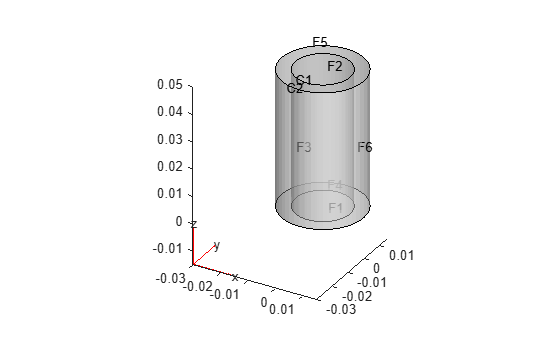Specify Young's modulus and Poisson's ratio for each metal.

```structuralProperties(structuralmodel,"Cell",1,"YoungsModulus",110E9, ... "PoissonsRatio",0.28); structuralProperties(structuralmodel,"Cell",2,"YoungsModulus",210E9, ... "PoissonsRatio",0.3);```

Specify that faces 1 and 4 are fixed boundaries.

`structuralBC(structuralmodel,"Face",[1,4],"Constraint","fixed");`

Specify the surface traction for faces 2 and 5.

```structuralBoundaryLoad(structuralmodel,"Face",[2,5], ... "SurfaceTraction",[0;0;100]);```

Generate a mesh and solve the problem.

```generateMesh(structuralmodel); structuralresults = solve(structuralmodel)```
```structuralresults = StaticStructuralResults with properties: Displacement: [1x1 FEStruct] Strain: [1x1 FEStruct] Stress: [1x1 FEStruct] VonMisesStress: [22402x1 double] Mesh: [1x1 FEMesh] ```

The solver finds the values of the displacement, stress, strain, and von Mises stress at the nodal locations. To access these values, use `structuralresults.Displacement`, `structuralresults.Stress`, and so on. The displacement, stress, and strain values at the nodal locations are returned as `FEStruct` objects with the properties representing their components. Note that properties of an `FEStruct` object are read-only.

`structuralresults.Displacement`
```ans = FEStruct with properties: ux: [22402x1 double] uy: [22402x1 double] uz: [22402x1 double] Magnitude: [22402x1 double] ```
`structuralresults.Stress`
```ans = FEStruct with properties: sxx: [22402x1 double] syy: [22402x1 double] szz: [22402x1 double] syz: [22402x1 double] sxz: [22402x1 double] sxy: [22402x1 double] ```
`structuralresults.Strain`
```ans = FEStruct with properties: exx: [22402x1 double] eyy: [22402x1 double] ezz: [22402x1 double] eyz: [22402x1 double] exz: [22402x1 double] exy: [22402x1 double] ```

Plot the deformed shape with the z-component of normal stress.

```pdeplot3D(structuralmodel, ... "ColorMapData",structuralresults.Stress.szz, ... "Deformation",structuralresults.Displacement)```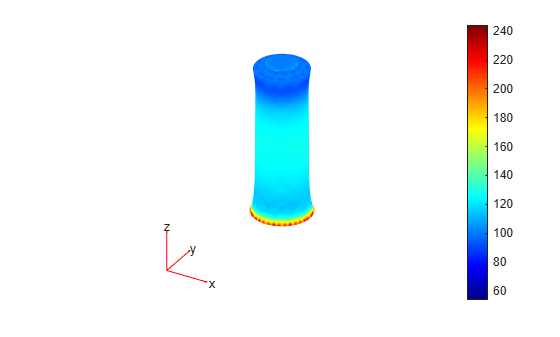Solve for the transient response of a thin 3-D plate under a harmonic load at the center.

Create a transient dynamic model for a 3-D problem.

`structuralmodel = createpde("structural","transient-solid");`

Create the geometry and include it in the model. Plot the geometry.

```gm = multicuboid([5,0.05],[5,0.05],0.01); structuralmodel.Geometry = gm; pdegplot(structuralmodel,"FaceLabels","on","FaceAlpha",0.5)```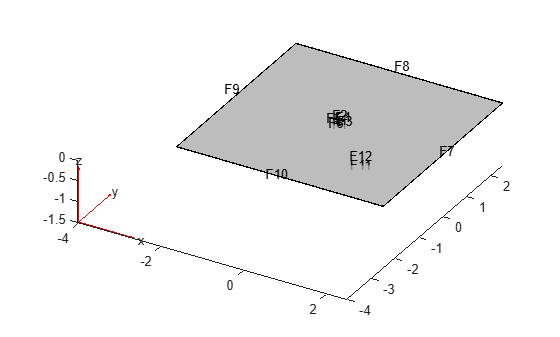Zoom in to see the face labels on the small plate at the center.

```figure pdegplot(structuralmodel,"FaceLabels","on","FaceAlpha",0.25) axis([-0.2 0.2 -0.2 0.2 -0.1 0.1])```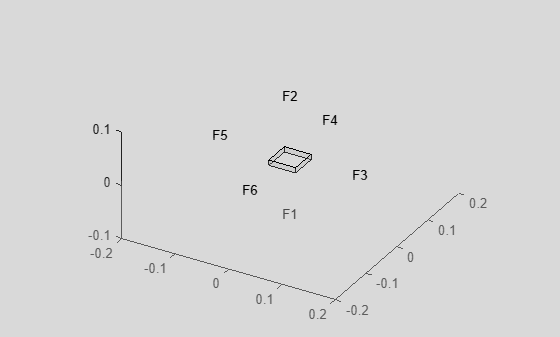Specify Young's modulus, Poisson's ratio, and the mass density of the material.

```structuralProperties(structuralmodel,"YoungsModulus",210E9, ... "PoissonsRatio",0.3, ... "MassDensity",7800);```

Specify that all faces on the periphery of the thin 3-D plate are fixed boundaries.

`structuralBC(structuralmodel,"Constraint","fixed","Face",5:8);`

Apply a sinusoidal pressure load on the small face at the center of the plate.

```structuralBoundaryLoad(structuralmodel,"Face",12, ... "Pressure",5E7, ... "Frequency",25);```

Generate a mesh with linear elements.

`generateMesh(structuralmodel,"GeometricOrder","linear","Hmax",0.2);`

Specify zero initial displacement and velocity.

`structuralIC(structuralmodel,"Displacement",[0;0;0],"Velocity",[0;0;0]);`

Solve the model.

```tlist = linspace(0,1,300); structuralresults = solve(structuralmodel,tlist);```

The solver finds the values of the displacement, velocity, and acceleration at the nodal locations. To access these values, use `structuralresults.Displacement`, `structuralresults.Velocity`, and so on. The displacement, velocity, and acceleration values are returned as `FEStruct` objects with the properties representing their components. Note that properties of an `FEStruct` object are read-only.

`structuralresults.Displacement`
```ans = FEStruct with properties: ux: [1873x300 double] uy: [1873x300 double] uz: [1873x300 double] Magnitude: [1873x300 double] ```
`structuralresults.Velocity`
```ans = FEStruct with properties: vx: [1873x300 double] vy: [1873x300 double] vz: [1873x300 double] Magnitude: [1873x300 double] ```
`structuralresults.Acceleration`
```ans = FEStruct with properties: ax: [1873x300 double] ay: [1873x300 double] az: [1873x300 double] Magnitude: [1873x300 double] ```

Perform frequency response analysis of a tuning fork.

First, create a structural model for modal analysis of a solid tuning fork.

`model = createpde("structural","frequency-solid");`

Import the tuning fork geometry.

`importGeometry(model,"TuningFork.stl");`

Specify Young's modulus, Poisson's ratio, and the mass density to model linear elastic material behavior. Specify all physical properties in consistent units.

```structuralProperties(model,"YoungsModulus",210E9, ... "PoissonsRatio",0.3, ... "MassDensity",8000);```

Identify faces for applying boundary constraints and loads by plotting the geometry with the face labels.

```figure("units","normalized","outerposition",[0 0 1 1]) pdegplot(model,"FaceLabels","on") view(-50,15) title("Geometry with Face Labels")```Impose sufficient boundary constraints to prevent rigid body motion under applied loading. Typically, you hold a tuning fork by hand or mount it on a table. To create a simple approximation of this boundary condition, fix a region near the intersection of tines and the handle (faces 21 and 22).

`structuralBC(model,"Face",[21,22],"Constraint","fixed");`

Specify the pressure loading on a tine (face 11) as a short rectangular pressure pulse. In the frequency domain, this pressure pulse is a unit load uniformly distributed across all frequencies.

```structuralBoundaryLoad(model,"Face",11,"Pressure",1); flist = linspace(0,4000,150); mesh = generateMesh(model,"Hmax",0.005); R = solve(model,2*pi*flist);```

Plot the vibration frequency of the tine tip, which is face 12. Find nodes on the tip face and plot the y-component of the displacement over the frequency, using one of these nodes.

```excitedTineTipNodes = findNodes(mesh,"region","Face",12); tipDisp = R.Displacement.uy(excitedTineTipNodes(1),:); figure plot(flist,abs(tipDisp)) xlabel("Frequency"); ylabel("|Y-Displacement|");```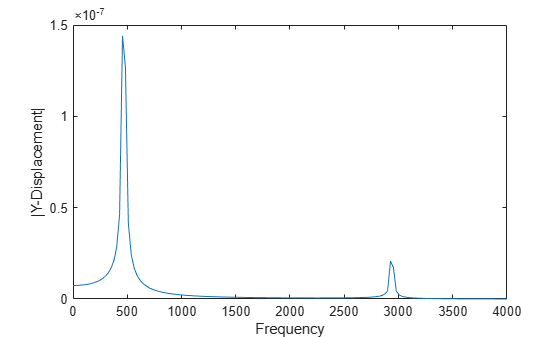Find the fundamental (lowest) mode of a 2-D cantilevered beam, assuming prevalence of the plane-stress condition.

Specify geometric and structural properties of the beam, along with a unit plane-stress thickness.

```length = 5; height = 0.1; E = 3E7; nu = 0.3; rho = 0.3/386;```

Create a modal plane-stress model, assign a geometry, and generate a mesh.

```structuralmodel = createpde("structural","modal-planestress"); gdm = [3;4;0;length;length;0;0;0;height;height]; g = decsg(gdm,'S1',('S1')'); geometryFromEdges(structuralmodel,g);```

Define a maximum element size (five elements through the beam thickness).

```hmax = height/5; msh=generateMesh(structuralmodel,"Hmax",hmax);```

Specify the structural properties and boundary constraints.

```structuralProperties(structuralmodel,"YoungsModulus",E, ... "MassDensity",rho, ... "PoissonsRatio",nu); structuralBC(structuralmodel,"Edge",4,"Constraint","fixed");```

Compute the analytical fundamental frequency (Hz) using the beam theory.

```I = height^3/12; analyticalOmega1 = 3.516*sqrt(E*I/(length^4*(rho*height)))/(2*pi)```
```analyticalOmega1 = 126.9498 ```

Specify a frequency range that includes an analytically computed frequency and solve the model.

`modalresults = solve(structuralmodel,"FrequencyRange",[0,1e6])`
```modalresults = ModalStructuralResults with properties: NaturalFrequencies: [32x1 double] ModeShapes: [1x1 FEStruct] Mesh: [1x1 FEMesh] ```

The solver finds natural frequencies and modal displacement values at nodal locations. To access these values, use `modalresults.NaturalFrequencies` and `modalresults.ModeShapes`.

`modalresults.NaturalFrequencies/(2*pi)`
```ans = 32×1 105 × 0.0013 0.0079 0.0222 0.0433 0.0711 0.0983 0.1055 0.1462 0.1930 0.2455 ⋮ ```
`modalresults.ModeShapes`
```ans = FEStruct with properties: ux: [6511x32 double] uy: [6511x32 double] Magnitude: [6511x32 double] ```

Plot the y-component of the solution for the fundamental frequency.

```pdeplot(structuralmodel,"XYData",modalresults.ModeShapes.uy(:,1)) title(['First Mode with Frequency ', ... num2str(modalresults.NaturalFrequencies(1)/(2*pi)),' Hz']) axis equal```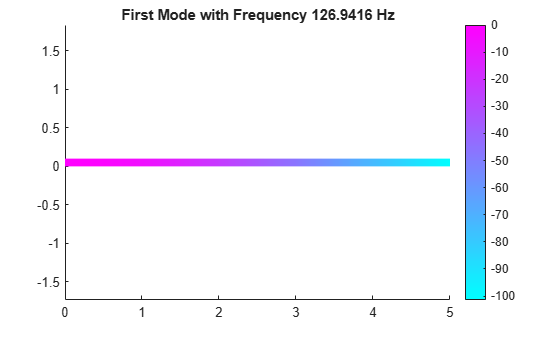Solve for the transient response at the center of a 3-D beam under a harmonic load on one of its corners.

Modal Analysis

Create a modal analysis model for a 3-D problem.

`structuralmodel = createpde("structural","modal-solid");`

Create the geometry and include it in the model. Plot the geometry and display the edge and vertex labels.

```gm = multicuboid(0.05,0.003,0.003); structuralmodel.Geometry = gm; pdegplot(structuralmodel,"EdgeLabels","on", ... "VertexLabels","on"); view([95 5])```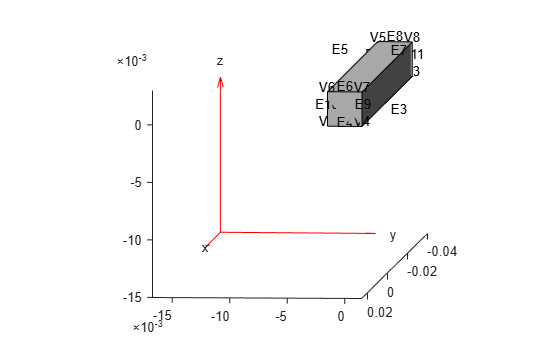Generate a mesh.

`msh = generateMesh(structuralmodel);`

Specify Young's modulus, Poisson's ratio, and the mass density of the material.

```structuralProperties(structuralmodel,"YoungsModulus",210E9, ... "PoissonsRatio",0.3, ... "MassDensity",7800);```

Specify minimal constraints on one end of the beam to prevent rigid body modes. For example, specify that edge 4 and vertex 7 are fixed boundaries.

```structuralBC(structuralmodel,"Edge",4,"Constraint","fixed"); structuralBC(structuralmodel,"Vertex",7,"Constraint","fixed");```

Solve the problem for the frequency range from 0 to 500,000. The recommended approach is to use a value that is slightly lower than the expected lowest frequency. Thus, use `-0.1` instead of `0`.

`Rm = solve(structuralmodel,"FrequencyRange",[-0.1,500000]);`

Transient Analysis

Switch the analysis type of the model to transient.

`structuralmodel.AnalysisType = "transient-solid";`

Apply a sinusoidal force on the corner opposite of the constrained edge and vertex.

```structuralBoundaryLoad(structuralmodel,"Vertex",5, ... "Force",[0,0,10], ... "Frequency",7600);```

Specify zero initial displacement and velocity.

```structuralIC(structuralmodel,"Velocity",[0;0;0], ... "Displacement",[0;0;0]);```

Specify the relative and absolute tolerances for the solver.

```structuralmodel.SolverOptions.RelativeTolerance = 1E-5; structuralmodel.SolverOptions.AbsoluteTolerance = 1E-9;```

Solve the model using the modal results.

```tlist = linspace(0,0.004,120); Rdm = solve(structuralmodel,tlist,"ModalResults",Rm);```

Interpolate and plot the displacement at the center of the beam.

```intrpUdm = interpolateDisplacement(Rdm,0,0,0.0015); plot(Rdm.SolutionTimes,intrpUdm.uz) grid on xlabel("Time"); ylabel("Center of beam displacement")```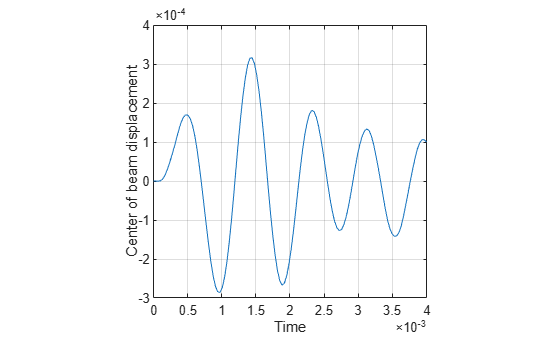Find the deflection of a 3-D cantilever beam under a nonuniform thermal load. Specify the thermal load on the structural model using the solution from a transient thermal analysis on the same geometry and mesh.

Transient Thermal Model Analysis

Create a transient thermal model.

`thermalmodel = createpde("thermal","transient");`

Create and plot the geometry.

```gm = multicuboid(0.5,0.1,0.05); thermalmodel.Geometry = gm; pdegplot(thermalmodel,"FaceLabels","on","FaceAlpha",0.5)```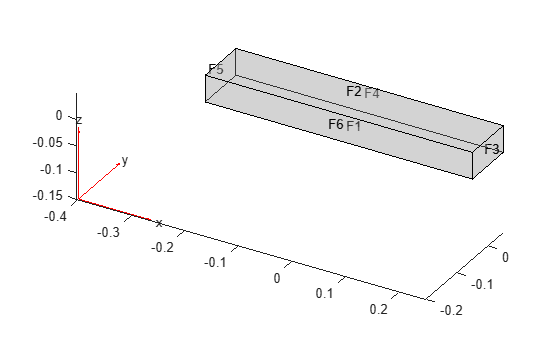Generate a mesh.

`mesh = generateMesh(thermalmodel);`

Specify the thermal properties of the material.

```thermalProperties(thermalmodel,"ThermalConductivity",5e-3, ... "MassDensity",2.7*10^(-6), ... "SpecificHeat",10);```

Specify the constant temperatures applied to the left and right ends of the beam.

```thermalBC(thermalmodel,"Face",3,"Temperature",100); thermalBC(thermalmodel,"Face",5,"Temperature",0);```

Specify the heat source over the entire geometry.

`internalHeatSource(thermalmodel,10);`

Set the initial temperature.

`thermalIC(thermalmodel,0);`

Solve the model.

```tlist = [0:1e-4:2e-4]; thermalresults = solve(thermalmodel,tlist)```
```thermalresults = TransientThermalResults with properties: Temperature: [3870x3 double] SolutionTimes: [0 1.0000e-04 2.0000e-04] XGradients: [3870x3 double] YGradients: [3870x3 double] ZGradients: [3870x3 double] Mesh: [1x1 FEMesh] ```

Plot the temperature distribution for each time step.

```for n = 1:numel(thermalresults.SolutionTimes) figure pdeplot3D(thermalmodel, ... "ColorMapData", ... thermalresults.Temperature(:,n)) title(["Temperature at Time = " ... num2str(tlist(n))]) caxis([0 100]) end```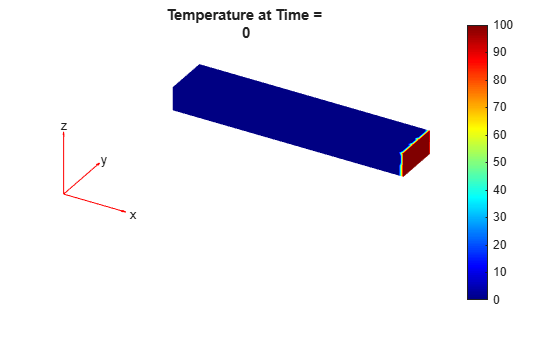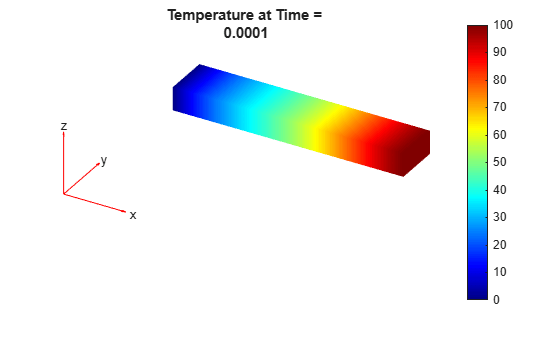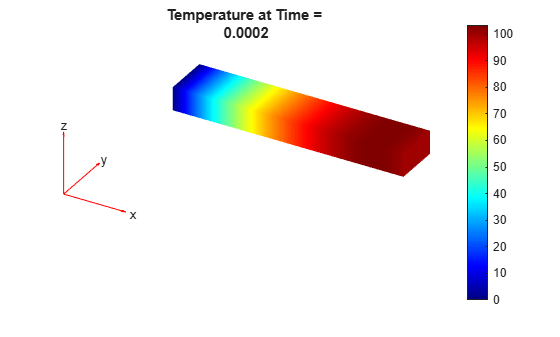Create a static structural model.

`structuralmodel = createpde("structural","static-solid");`

Include the same geometry as for the thermal model.

`structuralmodel.Geometry = gm;`

Use the same mesh that you used to obtain the thermal solution.

`structuralmodel.Mesh = mesh;`

Specify Young's modulus, Poisson's ratio, and the coefficient of thermal expansion.

```structuralProperties(structuralmodel,"YoungsModulus",1e10, ... "PoissonsRatio",0.3, ... "CTE",11.7e-6);```

Apply a fixed boundary condition on face 5.

`structuralBC(structuralmodel,"Face",5,"Constraint","fixed");`

Apply a body load using the transient thermal model solution. By default, `structuralBodyLoad` uses the solution for the last time step.

`structuralBodyLoad(structuralmodel,"Temperature",thermalresults);`

Specify the reference temperature.

`structuralmodel.ReferenceTemperature = 10;`

Solve the structural model.

`thermalstressresults = solve(structuralmodel);`

Plot the deformed shape of the beam corresponding to the last step of the transient thermal model solution.

```pdeplot3D(structuralmodel, ... "ColorMapData", ... thermalstressresults.Displacement.Magnitude, ... "Deformation", ... thermalstressresults.Displacement) title(["Thermal Expansion at Solution Time = " ... num2str(tlist(end))]) caxis([0 3e-3])```Now specify the body loads as the thermal model solutions for all time steps. For each body load, solve the structural model and plot the corresponding deformed shape of the beam.

```for n = 1:numel(thermalresults.SolutionTimes) structuralBodyLoad(structuralmodel, ... "Temperature", ... thermalresults, ... "TimeStep",n); thermalstressresults = solve(structuralmodel); figure pdeplot3D(structuralmodel, ... "ColorMapData", ... thermalstressresults.Displacement.Magnitude, ... "Deformation", ... thermalstressresults.Displacement) title(["Thermal Results at Solution Time = " ... num2str(tlist(n))]) caxis([0 3e-3]) end```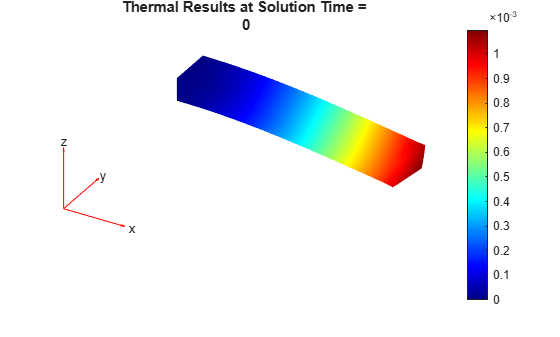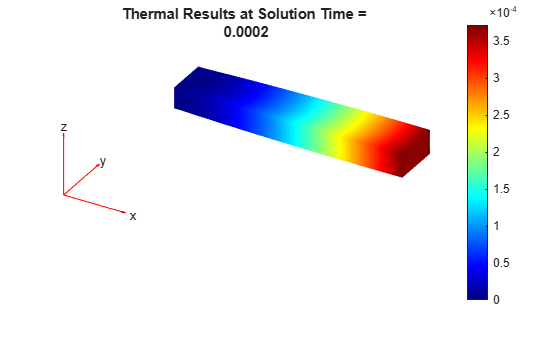Solve a 3-D steady-state thermal problem.

Create a thermal model for this problem.

`thermalmodel = createpde("thermal");`

Import and plot the block geometry.

```importGeometry(thermalmodel,"Block.stl"); pdegplot(thermalmodel,"FaceLabel","on","FaceAlpha",0.5) axis equal```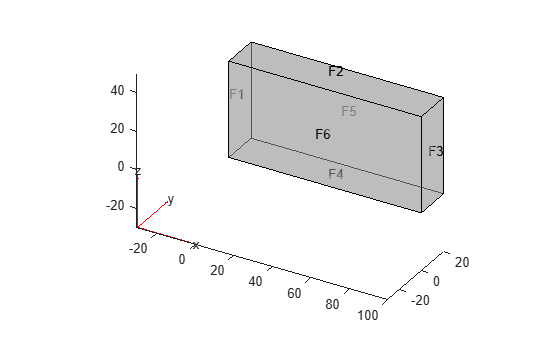Assign material properties.

`thermalProperties(thermalmodel,"ThermalConductivity",80);`

Apply a constant temperature of 100 °C to the left side of the block (face 1) and a constant temperature of 300 °C to the right side of the block (face 3). All other faces are insulated by default.

```thermalBC(thermalmodel,"Face",1,"Temperature",100); thermalBC(thermalmodel,"Face",3,"Temperature",300);```

Mesh the geometry and solve the problem.

```generateMesh(thermalmodel); thermalresults = solve(thermalmodel)```
```thermalresults = SteadyStateThermalResults with properties: Temperature: [12691x1 double] XGradients: [12691x1 double] YGradients: [12691x1 double] ZGradients: [12691x1 double] Mesh: [1x1 FEMesh] ```

The solver finds the temperatures and temperature gradients at the nodal locations. To access these values, use `thermalresults.Temperature`, `thermalresults.XGradients`, and so on. For example, plot temperatures at the nodal locations.

`pdeplot3D(thermalmodel,"ColorMapData",thermalresults.Temperature)`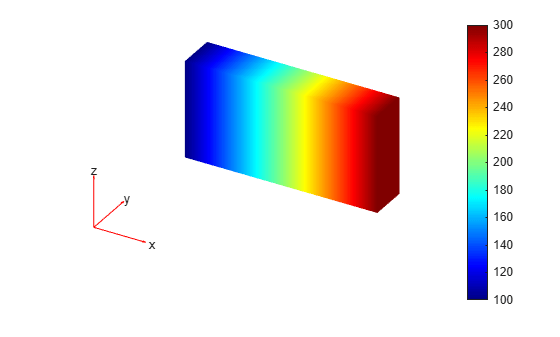Solve a 2-D transient thermal problem.

Create a transient thermal model for this problem.

`thermalmodel = createpde("thermal","transient");`

Create the geometry and include it in the model.

```SQ1 = [3; 4; 0; 3; 3; 0; 0; 0; 3; 3]; D1 = [2; 4; 0.5; 1.5; 2.5; 1.5; 1.5; 0.5; 1.5; 2.5]; gd = [SQ1 D1]; sf = 'SQ1+D1'; ns = char('SQ1','D1'); ns = ns'; dl = decsg(gd,sf,ns); geometryFromEdges(thermalmodel,dl); pdegplot(thermalmodel,"EdgeLabels","on","FaceLabels","on") xlim([-1.5 4.5]) ylim([-0.5 3.5]) axis equal```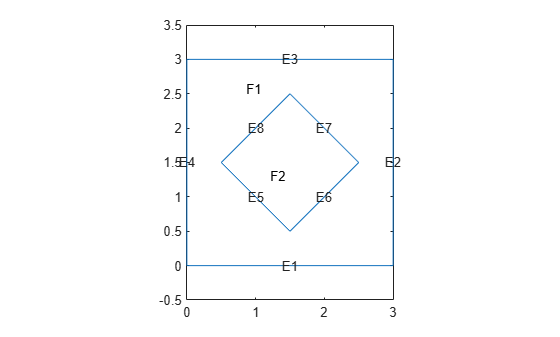For the square region, assign these thermal properties:

• Thermal conductivity is $10\text{\hspace{0.17em}}\mathrm{W}/\left(\mathrm{m}{\cdot }^{\circ }\mathrm{C}\right)$

• Mass density is $2\text{\hspace{0.17em}}\mathrm{kg}/{\mathrm{m}}^{3}$

• Specific heat is $0.1\text{\hspace{0.17em}}\mathrm{J}/\left({\mathrm{kg}\cdot }^{\circ }\mathrm{C}\right)$

```thermalProperties(thermalmodel,"ThermalConductivity",10, ... "MassDensity",2, ... "SpecificHeat",0.1, ... "Face",1);```

For the diamond region, assign these thermal properties:

• Thermal conductivity is $2\text{\hspace{0.17em}}\mathrm{W}/\left(\mathrm{m}{\cdot }^{\circ }\mathrm{C}\right)$

• Mass density is $1\text{\hspace{0.17em}}\mathrm{kg}/{\mathrm{m}}^{3}$

• Specific heat is $0.1\text{\hspace{0.17em}}\mathrm{J}/\left({\mathrm{kg}\cdot }^{\circ }\mathrm{C}\right)$

```thermalProperties(thermalmodel,"ThermalConductivity",2, ... "MassDensity",1, ... "SpecificHeat",0.1, ... "Face",2);```

Assume that the diamond-shaped region is a heat source with a density of $4\text{\hspace{0.17em}}\mathrm{W}/{\mathrm{m}}^{2}$.

`internalHeatSource(thermalmodel,4,"Face",2);`

Apply a constant temperature of 0 °C to the sides of the square plate.

`thermalBC(thermalmodel,"Temperature",0,"Edge",[1 2 7 8]);`

Set the initial temperature to 0 °C.

`thermalIC(thermalmodel,0);`

Generate the mesh.

`generateMesh(thermalmodel);`

The dynamics for this problem are very fast. The temperature reaches a steady state in about 0.1 second. To capture the most active part of the dynamics, set the solution time to `logspace(-2,-1,10)`. This command returns 10 logarithmically spaced solution times between 0.01 and 0.1.

`tlist = logspace(-2,-1,10);`

Solve the equation.

`thermalresults = solve(thermalmodel,tlist);`

Plot the solution with isothermal lines by using a contour plot.

```T = thermalresults.Temperature; pdeplot(thermalmodel,"XYData",T(:,10),"Contour","on","ColorMap","hot")```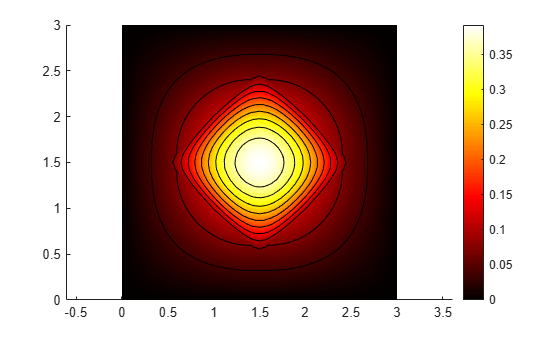Solve a transient thermal problem by first obtaining mode shapes for a particular decay range and then using the modal superposition method.

Modal Decomposition

First, create a modal thermal model.

`thermalmodel = createpde("thermal","modal");`

Create the geometry and include it in the model.

```SQ1 = [3; 4; 0; 3; 3; 0; 0; 0; 3; 3]; D1 = [2; 4; 0.5; 1.5; 2.5; 1.5; 1.5; 0.5; 1.5; 2.5]; gd = [SQ1 D1]; sf = 'SQ1+D1'; ns = char('SQ1','D1'); ns = ns'; dl = decsg(gd,sf,ns); geometryFromEdges(thermalmodel,dl); pdegplot(thermalmodel,"EdgeLabels","on","FaceLabels","on") xlim([-1.5 4.5]) ylim([-0.5 3.5]) axis equal```For the square region, assign these thermal properties:

• Thermal conductivity is $10\text{\hspace{0.17em}}\mathrm{W}/\left(\mathrm{m}{\cdot }^{\circ }\mathrm{C}\right)$.

• Mass density is $2\text{\hspace{0.17em}}\mathrm{kg}/{\mathrm{m}}^{3}$.

• Specific heat is $0.1\text{\hspace{0.17em}}\mathrm{J}/\left({\mathrm{kg}\cdot }^{\circ }\mathrm{C}\right)$.

```thermalProperties(thermalmodel,"ThermalConductivity",10, ... "MassDensity",2, ... "SpecificHeat",0.1, ... "Face",1);```

For the diamond region, assign these thermal properties:

• Thermal conductivity is $2\text{\hspace{0.17em}}\mathrm{W}/\left(\mathrm{m}{\cdot }^{\circ }\mathrm{C}\right)$.

• Mass density is $1\text{\hspace{0.17em}}\mathrm{kg}/{\mathrm{m}}^{3}$.

• Specific heat is $0.1\text{\hspace{0.17em}}\mathrm{J}/\left({\mathrm{kg}\cdot }^{\circ }\mathrm{C}\right)$.

```thermalProperties(thermalmodel,"ThermalConductivity",2, ... "MassDensity",1, ... "SpecificHeat",0.1, ... "Face",2);```

Assume that the diamond-shaped region is a heat source with a density of $4\text{\hspace{0.17em}}\mathrm{W}/{\mathrm{m}}^{2}$.

`internalHeatSource(thermalmodel,4,"Face",2);`

Apply a constant temperature of 0 °C to the sides of the square plate.

`thermalBC(thermalmodel,"Temperature",0,"Edge",[1 2 7 8]);`

Set the initial temperature to 0 °C.

`thermalIC(thermalmodel,0);`

Generate the mesh.

`generateMesh(thermalmodel);`

Compute eigenmodes of the thermal model in the decay range [100,10000] ${s}^{-1}$.

`RModal = solve(thermalmodel,"DecayRange",[100,10000])`
```RModal = ModalThermalResults with properties: DecayRates: [164x1 double] ModeShapes: [1481x164 double] ModeType: "EigenModes" Mesh: [1x1 FEMesh] ```

Transient Analysis

Knowing the mode shapes, you can now use the modal superposition method to solve the transient thermal problem. First, switch the thermal model analysis type to transient.

`thermalmodel.AnalysisType = "transient";`

The dynamics for this problem are very fast. The temperature reaches a steady state in about 0.1 second. To capture the most active part of the dynamics, set the solution time to `logspace(-2,-1,100)`. This command returns 100 logarithmically spaced solution times between 0.01 and 0.1.

`tlist = logspace(-2,-1,10);`

Solve the equation.

`Rtransient = solve(thermalmodel,tlist,"ModalResults",RModal);`

Plot the solution with isothermal lines by using a contour plot.

```T = Rtransient.Temperature; pdeplot(thermalmodel,"XYData",T(:,end), ... "Contour","on", ... "ColorMap","hot")```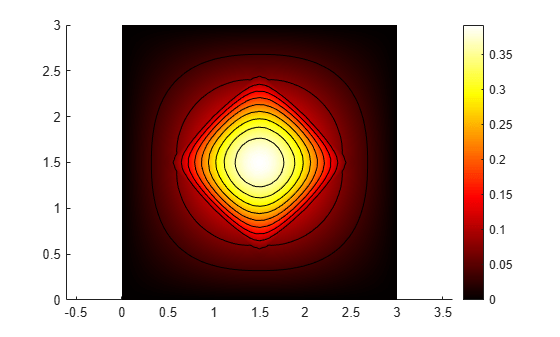Obtain POD modes of a linear thermal model using several instances of the transient solution (snapshots).

Create a transient thermal model.

`thermalmodel = createpde("thermal","transient");`

Create a unit square geometry and include it in the model.

`geometryFromEdges(thermalmodel,@squareg);`

Plot the geometry, displaying edge labels.

```pdegplot(thermalmodel,"EdgeLabels","on") xlim([-1.1 1.1]) ylim([-1.1 1.1])```Specify the thermal conductivity, mass density, and specific heat of the material.

```thermalProperties(thermalmodel,"ThermalConductivity",400, ... "MassDensity",1300, ... "SpecificHeat",600);```

Set the temperature on the right edge to `100`.

`thermalBC(thermalmodel,"Edge",2,"Temperature",100);`

Set an initial value of `0` for the temperature.

`thermalIC(thermalmodel,0);`

Generate a mesh.

`generateMesh(thermalmodel);`

Solve the model for three different values of heat source and collect snapshots.

```tlist = 0:10:600; snapShotIDs = [1:10 59 60 61]; Tmatrix = []; heatVariation = [10000 15000 20000]; for q = heatVariation internalHeatSource(thermalmodel,q); results = solve(thermalmodel,tlist); Tmatrix = [Tmatrix,results.Temperature(:,snapShotIDs)]; end```

Switch the thermal model analysis type to modal.

`thermalmodel.AnalysisType = "modal";`

Compute the POD modes.

`RModal = solve(thermalmodel,"Snapshots",Tmatrix)`
```RModal = ModalThermalResults with properties: DecayRates: [6x1 double] ModeShapes: [1541x6 double] SnapshotsAverage: [1541x1 double] ModeType: "PODModes" Mesh: [1x1 FEMesh] ```

Solve an electromagnetic problem and find the electric potential and field distribution for a 2-D geometry representing a plate with a hole.

Create an electromagnetic model for electrostatic analysis.

`emagmodel = createpde("electromagnetic","electrostatic");`

Import and plot the geometry representing a plate with a hole.

```importGeometry(emagmodel,"PlateHolePlanar.stl"); pdegplot(emagmodel,"EdgeLabels","on")```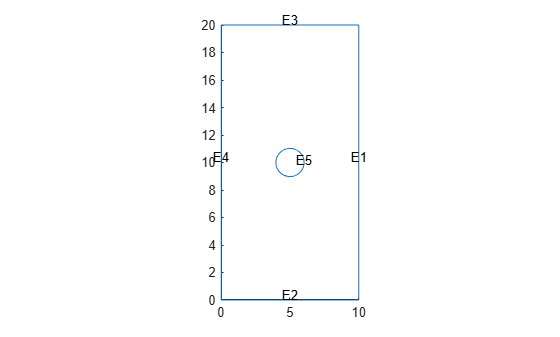Specify the vacuum permittivity value in the SI system of units.

`emagmodel.VacuumPermittivity = 8.8541878128E-12;`

Specify the relative permittivity of the material.

`electromagneticProperties(emagmodel,"RelativePermittivity",1);`

Apply the voltage boundary conditions on the edges framing the rectangle and the circle.

```electromagneticBC(emagmodel,"Voltage",0,"Edge",1:4); electromagneticBC(emagmodel,"Voltage",1000,"Edge",5);```

Specify the charge density for the entire geometry.

`electromagneticSource(emagmodel,"ChargeDensity",5E-9);`

Generate the mesh.

`generateMesh(emagmodel);`

Solve the model.

`R = solve(emagmodel)`
```R = ElectrostaticResults with properties: ElectricPotential: [1218x1 double] ElectricField: [1x1 FEStruct] ElectricFluxDensity: [1x1 FEStruct] Mesh: [1x1 FEMesh] ```

Plot the electric potential and field.

```pdeplot(emagmodel,"XYData",R.ElectricPotential, ... "FlowData",[R.ElectricField.Ex ... R.ElectricField.Ey]) axis equal```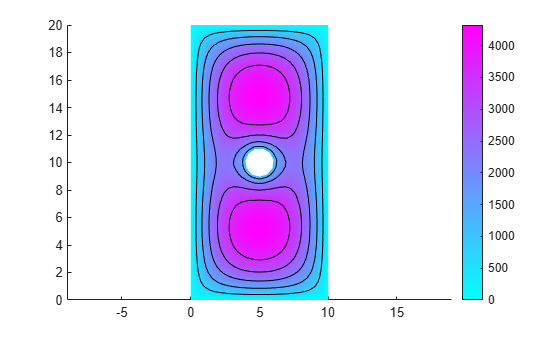Solve a 3-D electromagnetic problem on a geometry representing a plate with a hole in its center. Plot the resulting magnetic potential and field distribution.

Create an electromagnetic model for magnetostatic analysis.

`emagmodel = createpde("electromagnetic","magnetostatic");`

Import and plot the geometry representing a plate with a hole.

```importGeometry(emagmodel,"PlateHoleSolid.stl"); pdegplot(emagmodel,"FaceLabels","on","FaceAlpha",0.3)```Specify the vacuum permeability value in the SI system of units.

`emagmodel.VacuumPermeability = 1.2566370614e-6;`

Specify the relative permeability of the material.

`electromagneticProperties(emagmodel,"RelativePermeability",5000);`

Apply the magnetic potential boundary conditions on the side faces and the face bordering the hole.

```electromagneticBC(emagmodel,"MagneticPotential",[0;0;0],"Face",3:6); electromagneticBC(emagmodel,"MagneticPotential",[0;0;0.01],"Face",7);```

Specify the current density for the entire geometry.

`electromagneticSource(emagmodel,"CurrentDensity",[0;0;0.5]);`

Generate the mesh.

`generateMesh(emagmodel);`

Solve the model.

`R = solve(emagmodel)`
```R = MagnetostaticResults with properties: MagneticPotential: [1x1 FEStruct] MagneticField: [1x1 FEStruct] MagneticFluxDensity: [1x1 FEStruct] Mesh: [1x1 FEMesh] ```

Plot the z-component of the magnetic potential.

`pdeplot3D(emagmodel,"ColormapData",R.MagneticPotential.Az)`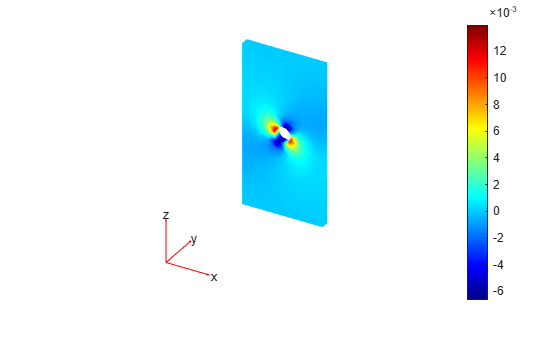Plot the magnetic field.

```pdeplot3D(emagmodel,"FlowData",[R.MagneticField.Hx ... R.MagneticField.Hy ... R.MagneticField.Hz])```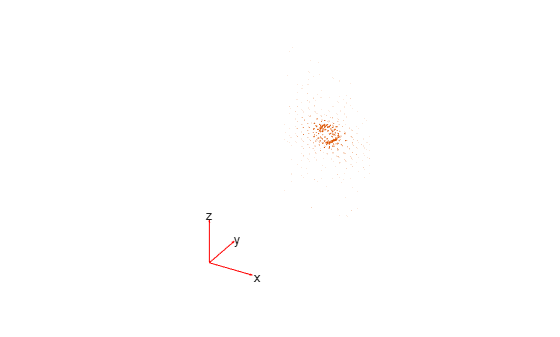Solve a DC conduction problem on a geometry representing a 3-D plate with a hole in its center. Plot the electric potential and the components of the current density.

Create an electromagnetic model for DC conduction analysis.

`emagmodel = createpde("electromagnetic","conduction");`

Import and plot a geometry representing a plate with a hole.

```gm = importGeometry(emagmodel,"PlateHoleSolid.stl"); pdegplot(gm,"FaceLabels","on","FaceAlpha",0.3)```Specify the conductivity of the material.

`electromagneticProperties(emagmodel,"Conductivity",6e4);`

Apply the voltage boundary conditions on the left, right, top, and bottom faces of the plate.

`electromagneticBC(emagmodel,"Voltage",0,"Face",3:6);`

Specify the surface current density on the face bordering the hole.

`electromagneticBC(emagmodel,"SurfaceCurrentDensity",100,"Face",7);`

Generate the mesh.

`generateMesh(emagmodel);`

Solve the model.

`R = solve(emagmodel)`
```R = ConductionResults with properties: ElectricPotential: [4359x1 double] ElectricField: [1x1 FEStruct] CurrentDensity: [1x1 FEStruct] Mesh: [1x1 FEMesh] ```

Plot the electric potential.

```figure pdeplot3D(emagmodel,"ColorMapData",R.ElectricPotential)```Plot the x-component of the current density.

```figure pdeplot3D(emagmodel,"ColorMapData",R.CurrentDensity.Jx) title("x-Component of Current Density")```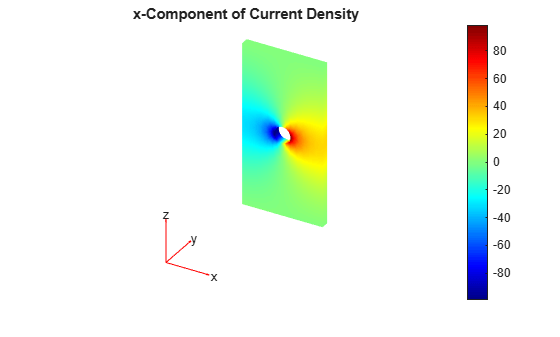Plot the y-component of the current density.

```figure pdeplot3D(emagmodel,"ColorMapData",R.CurrentDensity.Jy) title("y-Component of Current Density")```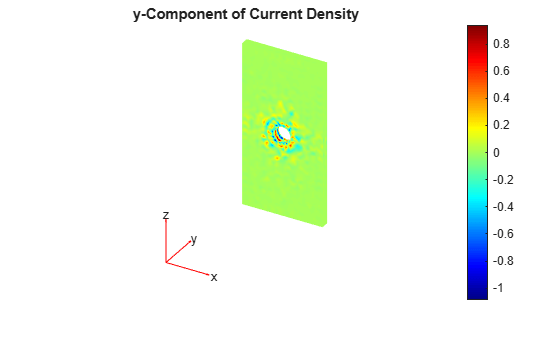Plot the z-component of the current density.

```figure pdeplot3D(emagmodel,"ColorMapData",R.CurrentDensity.Jz) title("z-Component of Current Density")```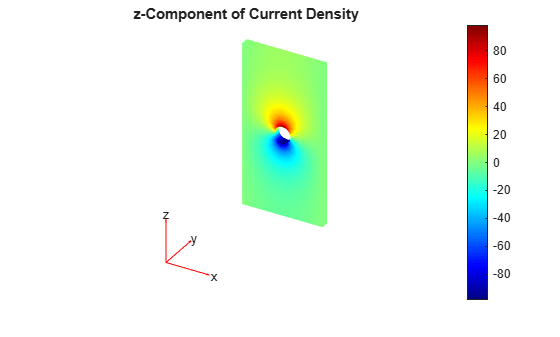Use a solution obtained by performing a DC conduction analysis to specify current density for a magnetostatic model.

Create an electromagnetic model for DC conduction analysis.

`emagmodel = createpde("electromagnetic","conduction");`

Import and plot a geometry representing a plate with a hole.

```gm = importGeometry(emagmodel,"PlateHoleSolid.stl"); pdegplot(gm,"FaceLabels","on","FaceAlpha",0.3)```Specify the conductivity of the material.

`electromagneticProperties(emagmodel,"Conductivity",6e4);`

Apply the voltage boundary conditions on the left, right, top, and bottom faces of the plate.

`electromagneticBC(emagmodel,"Voltage",0,"Face",3:6);`

Specify the surface current density on the face bordering the hole.

`electromagneticBC(emagmodel,"SurfaceCurrentDensity",100,"Face",7);`

Generate the mesh.

`generateMesh(emagmodel);`

Solve the model.

`R = solve(emagmodel);`

Change the analysis type of the model to magnetostatic.

`emagmodel.AnalysisType = "magnetostatic";`

This model already has a quadratic mesh that you generated for the DC conduction analysis. For a 3-D magnetostatic model, the mesh must be linear. Generate a new linear mesh. The `generateMesh` function creates a linear mesh by default if the model is 3-D and magnetostatic.

`generateMesh(emagmodel);`

Specify the vacuum permeability value in the SI system of units.

`emagmodel.VacuumPermeability = 1.2566370614e-6;`

Specify the relative permeability of the material.

`electromagneticProperties(emagmodel,"RelativePermeability",5000);`

Apply the magnetic potential boundary conditions on the side faces and the face bordering the hole.

```electromagneticBC(emagmodel,"MagneticPotential",[0;0;0],"Face",3:6); electromagneticBC(emagmodel,"MagneticPotential",[0;0;0.01],"Face",7);```

Specify the current density for the entire geometry using the DC conduction solution.

`electromagneticSource(emagmodel,"CurrentDensity",R);`

Solve the model.

`Rmagnetostatic = solve(emagmodel);`

Plot the x- and z-components of the magnetic potential.

`pdeplot3D(emagmodel,"ColormapData",Rmagnetostatic.MagneticPotential.Ax)`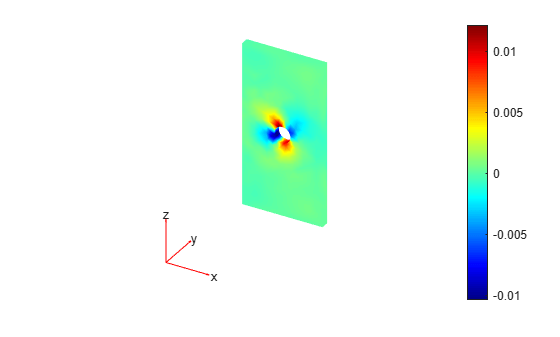`pdeplot3D(emagmodel,"ColormapData",Rmagnetostatic.MagneticPotential.Az)`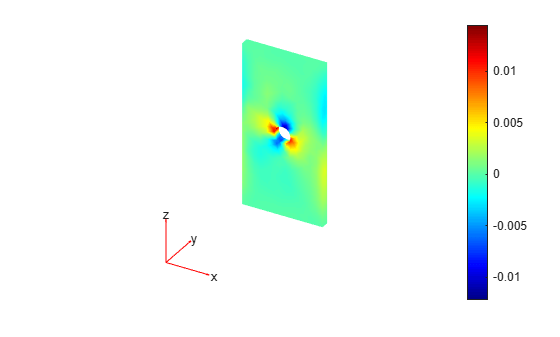Solve a magnetostatic model of a copper square with a permanent neodymium magnet in its center.

Create the unit square geometry with a circle in its center.

```L = 0.8; r = 0.25; sq = [3 4 -L L L -L -L -L L L]'; circ = [1 0 0 r 0 0 0 0 0 0]'; gd = [sq,circ]; sf = "sq + circ"; ns = char('sq','circ'); ns = ns'; g = decsg(gd,sf,ns);```

Plot the geometry with the face and edge labels.

`pdegplot(g,"FaceLabels","on","EdgeLabels","on")`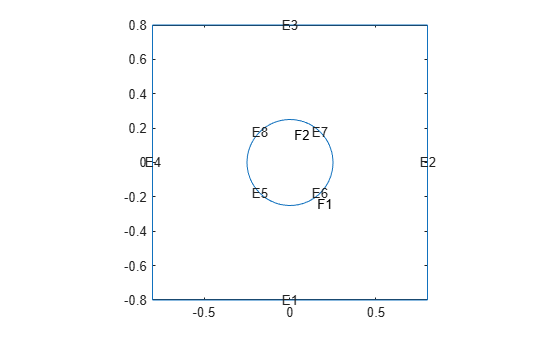Create a magnetostatic model and include the geometry in the model.

```emagmodel = createpde("electromagnetic","magnetostatic"); geometryFromEdges(emagmodel,g);```

Specify the vacuum permeability value in the SI system of units.

`emagmodel.VacuumPermeability = 1.2566370614e-6;`

Specify the relative permeability of the copper for the square.

```electromagneticProperties(emagmodel,"Face",1, ... "RelativePermeability",1);```

Specify the relative permeability of the neodymium for the circle.

```electromagneticProperties(emagmodel,"Face",2, ... "RelativePermeability",1.05);```

Specify the magnetization magnitude for the neodymium magnet.

`M = 1e6;`

Specify magnetization on the circular face in the positive x-direction. Magnetization for a 2-D model is a column vector of two elements.

```dir = [1;0]; electromagneticSource(emagmodel,"Face",2,"Magnetization",M*dir);```

Apply the magnetic potential boundary conditions on the edges framing the square.

`electromagneticBC(emagmodel,"Edge",1:4,"MagneticPotential",0);`

Generate the mesh with finer meshing near the edges of the circle.

```generateMesh(emagmodel,"Hedge",{5:8,0.007}); figure pdemesh(emagmodel)```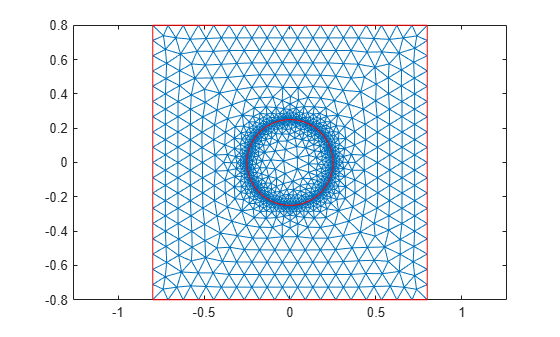Solve the model, and find the resulting magnetic fields B and H. Here, $\mathit{B}=\mu \mathit{H}+{\mu }_{0}\mathit{M}$, where $\mu$ is the absolute magnetic permeability of the material, ${\mu }_{0}$ is the vacuum permeability, and $\mathit{M}$ is the magnetization.

```R = solve(emagmodel); Bmag = sqrt(R.MagneticFluxDensity.Bx.^2 + R.MagneticFluxDensity.By.^2); Hmag = sqrt(R.MagneticField.Hx.^2 + R.MagneticField.Hy.^2);```

Plot the magnetic field B.

```figure pdeplot(emagmodel,"XYData",Bmag, ... "FlowData",[R.MagneticFluxDensity.Bx ... R.MagneticFluxDensity.By])```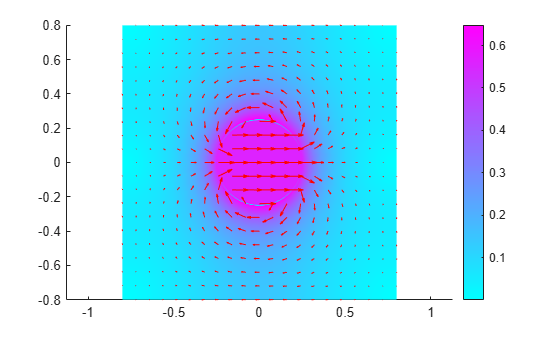Plot the magnetic field H.

```figure pdeplot(emagmodel,"XYData",Hmag, ... "FlowData",[R.MagneticField.Hx R.MagneticField.Hy])```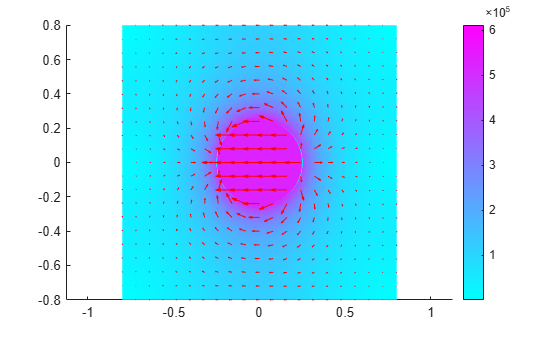For an electromagnetic harmonic analysis problem, find the x- and y-components of the electric field. Solve the problem on a domain consisting of a square with a circular hole.

Create an electromagnetic model for harmonic analysis.

`emagmodel = createpde("electromagnetic","harmonic");`

Define a circle in a square, place them in one matrix, and create a set formula that subtracts the circle from the square.

```SQ = [3,4,-5,-5,5,5,-5,5,5,-5]'; C = [1,0,0,1]'; C = [C;zeros(length(SQ) - length(C),1)]; gm = [SQ,C]; sf = 'SQ-C';```

Create the geometry.

```ns = char('SQ','C'); ns = ns'; g = decsg(gm,sf,ns);```

Include the geometry in the model and plot the geometry with the edge labels.

```geometryFromEdges(emagmodel,g); pdegplot(emagmodel,"EdgeLabels","on") xlim([-5.5 5.5]) ylim([-5.5 5.5])```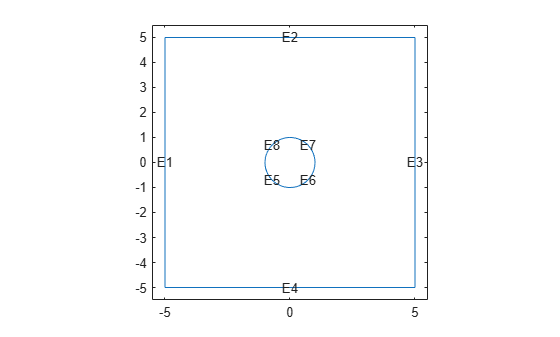Specify the vacuum permittivity and permeability values as 1.

```emagmodel.VacuumPermittivity = 1; emagmodel.VacuumPermeability = 1;```

Specify the relative permittivity, relative permeability, and conductivity of the material.

```electromagneticProperties(emagmodel,"RelativePermittivity",1, ... "RelativePermeability",1, ... "Conductivity",0);```

Apply the absorbing boundary condition with a thickness of 2 on the edges of the square. Use the default attenuation rate for the absorbing region.

```electromagneticBC(emagmodel,"Edge",1:4, ... "FarField","absorbing", ... "Thickness",2);```

Specify an electric field on the edges of the hole.

```E = @(location,state) [1;0]*exp(-1i*2*pi*location.y); electromagneticBC(emagmodel,"Edge",5:8,"ElectricField",E);```

Generate a mesh.

`generateMesh(emagmodel,"Hmax",1/2^3);`

Solve the model for a frequency of $2\pi$.

`result = solve(emagmodel,"Frequency",2*pi);`

Plot the real part of the x-component of the resulting electric field.

```figure pdeplot(emagmodel,"XYData",real(result.ElectricField.Ex)); title("Real Part of x-Component of Electric Field")```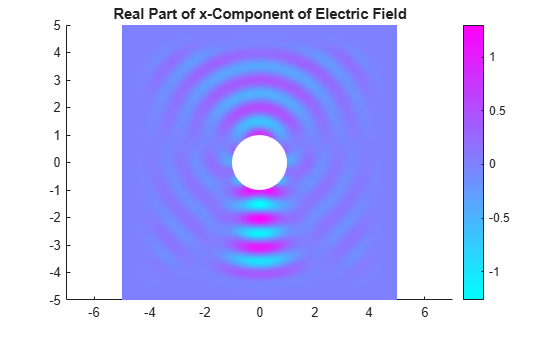Plot the real part of the y-component of the resulting electric field.

```figure pdeplot(emagmodel,"XYData",real(result.ElectricField.Ey)); title("Real Part of y-Component of Electric Field")```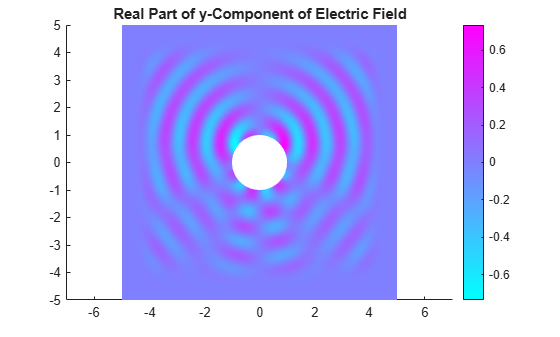## Input Arguments

collapse all

Static structural analysis model, specified as a `StructuralModel` object. The model contains the geometry, mesh, structural properties of the material, body loads, boundary loads, and boundary conditions.

Example: ```structuralmodel = createpde("structural","static-solid")```

Transient structural analysis model, specified as a `StructuralModel` object. The model contains the geometry, mesh, structural properties of the material, body loads, boundary loads, and boundary conditions.

Example: ```structuralmodel = createpde("structural","transient-solid")```

Frequency response analysis structural model, specified as a `StructuralModel` object. The model contains the geometry, mesh, structural properties of the material, body loads, boundary loads, and boundary conditions.

Example: ```structuralmodel = createpde("structural","frequency-solid")```

Modal analysis structural model, specified as a `StructuralModel` object. The model contains the geometry, mesh, structural properties of the material, body loads, boundary loads, and boundary conditions.

Example: ```structuralmodel = createpde("structural","modal-solid")```

Solution times for structural or thermal transient analysis, specified as a real vector of monotonically increasing or decreasing values.

Example: `0:20`

Data Types: `double`

Solution frequencies for a frequency response structural analysis, specified as a real vector of monotonically increasing or decreasing values.

Example: `linspace(0,4000,150)`

Data Types: `double`

Frequency range for a structural modal analysis, specified as a vector of two elements. Define `omega1` as slightly lower than the lowest expected frequency and `omega2` as slightly higher than the highest expected frequency. For example, if the lowest expected frequency is zero, then use a small negative value for `omega1`.

Example: `[-0.1,1000]`

Data Types: `double`

Modal analysis results for a structural model, specified as a `ModalStructuralResults` object.

Example: ```structuralModalR = solve(structuralmodel,"FrequencyRange",[0,1e6])```

Steady-state thermal analysis model, specified as a `ThermalModel` object. `ThermalModel` contains the geometry, mesh, thermal properties of the material, internal heat source, Stefan-Boltzmann constant, boundary conditions, and initial conditions.

Example: ```thermalmodel = createpde("thermal","steadystate")```

Transient thermal analysis model, specified as a `ThermalModel` object. `ThermalModel` contains the geometry, mesh, thermal properties of the material, internal heat source, Stefan-Boltzmann constant, boundary conditions, and initial conditions.

Modal thermal analysis model, specified as a `ThermalModel` object. `ThermalModel` contains the geometry, mesh, thermal properties of the material, internal heat source, Stefan-Boltzmann constant, boundary conditions, and initial conditions.

Decay range for modal thermal analysis, specified as a vector of two elements. The `solve` function solves a modal thermal analysis model for all modes in the decay range.

Data Types: `double`

Thermal model solution snapshots, specified as a matrix.

Data Types: `double`

Modal analysis results for a thermal model, specified as a `ModalThermalResults` object.

Example: ```thermalModalR = solve(thermalmodel,"DecayRange",[0,1000])```

Electromagnetic model for electrostatic, magnetostatic, or DC conduction analysis, specified as an `ElectromagneticModel` object. The model contains the geometry, mesh, material properties, electromagnetic sources, and boundary conditions.

Example: ```emagmodel = createpde("electromagnetic","magnetostatic")```

Solution frequencies for a harmonic electromagnetic analysis, specified as a nonnegative number or a vector of nonnegative numbers.

Data Types: `double`

## Output Arguments

collapse all

Static structural analysis results, returned as a `StaticStructuralResults` object.

Transient structural analysis results, returned as a `TransientStructuralResults` object.

Frequency response structural analysis results, returned as a `FrequencyStructuralResults` object.

Modal structural analysis results, returned as a `ModalStructuralResults` object.

Steady-state thermal analysis results, returned as a `SteadyStateThermalResults` object.

Transient thermal analysis results, returned as a `TransientThermalResults` object.

Modal thermal analysis results, returned as a `ModalThermalResults` object.

Electrostatic, magnetostatic, or DC conduction analysis results, returned as an `ElectrostaticResults`, `MagnetostaticResults`, or `ConductionResults` object.

Harmonic electromagnetic analysis results, returned as a `HarmonicResults` object.

## Tips

• When you use modal analysis results to solve a transient structural dynamics model, the `modalresults` argument must be created in Partial Differential Equation Toolbox™ from R2019a or newer.

• For a frequency response model with damping, the results are complex. Use functions such as `abs` and `angle` to obtain real-valued results, such as the magnitude and phase.

## Version History

Introduced in R2017a

expand all